Wind Energ. Sci., 7, 1693–1710, 2022
https://doi.org/10.5194/wes-7-1693-2022
Wind Energ. Sci., 7, 1693–1710, 2022
https://doi.org/10.5194/wes-7-1693-2022
Research article | 11 Aug 2022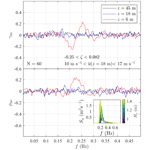# Turbulence in a coastal environment: the case of Vindeby

Turbulence in a coastal environment: the case of Vindeby
Rieska Mawarni Putria, Etienne Cheynetb, Charlotte Obhraia, and Jasna Bogunovic Jakobsena Rieska Mawarni Putri et al.
• aDepartment of Mechanical and Structural Engineering and Materials Science, University of Stavanger, 4036 Stavanger, Norway
• bGeophysical Institute and Bergen Offshore Wind Centre (BOW), University of Bergen, 5007 Bergen, Norway

Correspondence: Rieska Mawarni Putri (rieska.m.putri@uis.no)

Abstract

The one-point and two-point power spectral densities of the wind velocity fluctuations are studied using the observations from an offshore mast at Vindeby Offshore Wind Farm, for a wide range of thermal stratifications of the atmosphere. A comparison with estimates from the FINO1 platform (North Sea) is made to identify shared spectral characteristics of turbulence between different offshore sites. The sonic anemometer measurement data at 6, 18, and 45 m a.m.s.l. (above mean sea level) are considered. These heights are lower than at the FINO1 platform, where the measurements were collected at heights between 40 and 80 m. Although the sonic anemometers are affected by transducer-flow distortion, the spectra of the along-wind velocity component are consistent with those from FINO1 when surface-layer scaling is used, for near-neutral and moderately diabatic conditions. The co-coherence of the along-wind component, estimated for vertical separations under near-neutral conditions, matches remarkably well with the results from the dataset at the FINO1 platform. These findings mark an important step toward more comprehensive coherence models for wind load calculation. The turbulence characteristics estimated from the present dataset are valuable for better understanding the structure of turbulence in the marine atmospheric boundary layer and are relevant for load estimations of offshore wind turbines. Yet, the datasets recorded at Vindeby and FINO1 cover only the lower part of the rotor of state-of-the-art offshore wind turbines. Further improvements in the characterisation of atmospheric turbulence for wind turbine design will require measurements at heights above 100 m a.m.s.l.

Share
1 Introduction

In the early 1990s, the first generations of offshore wind farms were commissioned to test the viability of extracting wind power in the marine atmospheric boundary layer (MABL). The first was Vindeby Offshore Wind Farm, which provided electricity to around 2200 homes during its 25 years of operation, with a total generated power of 243 GW h . The project was deemed successful and marked the beginning of the offshore wind sector.

Not only was the Vindeby project the first offshore wind farm, but also it provided precious information on meteorological conditions in the MABL using offshore and onshore meteorological masts. The data collected have been used to study the characteristics of the mean wind speed profile under various atmospheric conditions . The masts were also instrumented with 3D sonic anemometers to study turbulence, but these data were used in a limited number of studies only (e.g.  Mahrt et al.1996, 2001).

The characteristics of the MABL differ from the overland atmospheric boundary layer (ABL) due to the large proportion of non-neutral atmospheric stability conditions and low roughness lengths. Since the 2010s, several studies have indicated that diabatic wind conditions may significantly affect the fatigue life of offshore wind turbine (OWT) components . Recent measurements from the first commercial floating wind farm (Hywind Scotland) have even shown the direct influence of atmospheric stability on the floater motions . Diabatic conditions are more likely to affect floating wind turbines than bottom-fixed ones as the first few eigenfrequencies of large floating wind turbines are close to or below 0.02 Hz , which is the frequency range mainly affected by the thermal stratification of the atmosphere. To model properly the wind load for wind turbine design, a better understanding of the spectral structure of turbulence in the MABL is necessary, which addresses partly the first of the three great challenges in the field of wind energy .

The limitations of current guidelines for offshore turbulence modelling, such as , have been highlighted in the past . Site-specific measurements advised by are related to the mean flow and integral turbulence characteristics. However, for the spectral characteristics, appropriate scaling can be used to display universal shapes over specific frequency ranges. In this regard, the present study addresses similar challenges to those discussed by but focuses on some specific aspects not covered by the spectral tensor of homogeneous turbulence (Mann1994). Firstly, the low-frequency fluctuations are generally underestimated by the uniform-shear model, especially under convective conditions . Secondly, the version of the uniform-shear model (Mann1994) used within the field of wind energy does not account for the blocking by the ground, which may lead to an overestimation of the co-coherence of both the along-wind and the vertical wind components for near-neutral conditions (Cheynet2019). Thus, the co-coherence modelling using the uniform-shear model (Mann1994) is not discussed further in the present study.

Using the unexplored sonic anemometer data from the Vindeby database, this study looks at the characteristics of offshore turbulence in the frequency space. The objective is to quantify the similarities between these characteristics and those identified at FINO1 . Such a comparison is relevant to establishing new offshore wind turbulence models that can be used to improve the design of future multi-megawatt offshore wind turbines. Whereas the measurement data from FINO1 were obtained 40 km away from the shore, at heights between 40 m and 80 m a.m.s.l. (above mean sea level), those from the Vindeby database were collected only 3 km from the coast and at heights between 6 m and 45 m a.m.s.l. Therefore, the two datasets offer a complementary description of wind turbulence above the sea.

The present study is organised as follows: Sect. 2 describes the instrumentation and the site topography. Section 3 summarises the data processing, the assumptions, and the models used to study the spectral characteristics of turbulence. Section 4 presents the methodology used to assess the data quality and selection of stationary velocity data. Section 5 first evaluates the applicability of surface-layer scaling for the anemometer records at 6 m a.m.s.l. Then, the one-point velocity spectra and co-coherence estimates from Vindeby are compared with predictions from semi-empirical models which are based on FINO1 observations to assess the similarities of the spectral characteristics between the two sites. Finally, the applicability of the Vindeby database to the design of an adequate turbulence model for offshore wind turbines is discussed in Sect. 5.5.

2 Instrumentation and site description

Vindeby Offshore Wind Farm operated in Denmark from 1991 to 2016 and was decommissioned in 2017. It was located 1.5 to 3 km from the northwestern coast of the island of Lolland (Fig. 1). Due to its location, Vindeby may be regarded as a coastal site instead of an offshore one. Vindeby has a flat topography with an average elevation of under 11 m a.m.s.l., whereas the water depth around the wind farm ranges from 2 to 5 m . As pointed out by , the average significant wave height Hs at Vindeby is under 1 m. The water depth increases from around 3 m in the proximity of the wind farm up to approximately 20 m away from the northern side of the wind farm.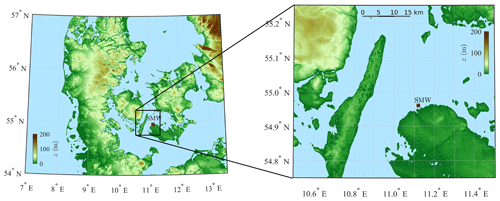Figure 1Digital elevation model of southern Denmark showing the location of Sea Mast West (SMW), in a sheltered flat coastal environment. Only the wind from 220 to 330 is considered for SMW.

The wind farm comprised 11 Bonus 450 kW turbines arranged in two rows with 300 m spacing along the 325–145 line and three meteorological masts (Fig. 2). The three masts were Land Mast (LM), Sea Mast South (SMS), and Sea Mast West (SMW), and the latter two were placed offshore (Fig. 2). Both SMS and SMW were installed in 1993 and were decommissioned in 2001 and 1998, respectively. Measurements from LM and SMS were used by to assess the influence of the thermal stratification of the atmosphere on coastal wind climates. The present study considers only wind measurements from SMW due to the availability of the data.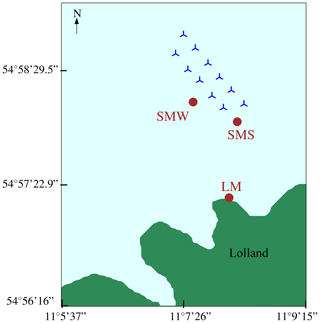Figure 2Vindeby Offshore Wind Farm layout with circles marking the position of the masts: SMW, SMS, and LM. SMW is located approximately 1.5 km from Lolland.

SMW was a triangular lattice tower with a height of 48 km a.m.s.l. as sketched in Fig. 3. The booms on the SMW were mounted on both sides of the tower at 46 and 226 from the north and are referred to as the northern and southern boom, respectively. The booms' length ranged from 1.6 to 4 m, and their diameter was 50 mm . Three F2360a Gill three-axis ultrasonic anemometers (SAs) were mounted on the southern booms at 45, 18, and 6 m a.m.s.l. and operated with a sampling rate of 20 Hz. Two Risø P2021 resolver wind vanes with P2058 wind direction transmitters were located on the northern booms at 43 m and 20 m a.m.s.l. using a sampling frequency of 5 Hz. The height of the vanes' centres above the boom was 600 mm. There were seven cup anemometers mounted on SMW as shown in Fig. 3; however, their measurements were not used here. The air temperature at 10 m a.m.s.l. was recorded using a Risø P2039 PT 100 sensor. The sea surface elevation η was measured using an acoustic wave recorder (AWR) placed on the seabed, 30 m away from SMW, at a depth of 4 m . The sea surface elevation data were recorded at a sampling frequency of 8 Hz but stored with a sampling frequency of 20 Hz. The data collected from SMW were transferred to LM using an underwater fibre optic link and stored as time series of 30 min duration. Such a duration is appropriate to study turbulence in coastal and offshore areas (Dobson1981). Therefore, the flow characteristics studied herein are based on the averaging time of 30 min.

The fetch around SMW comprises open sea, land, and mixed fetch as shown in Fig. 1. The so-called sea fetch is considered when the wind blows from 220 to 90, with a fetch distance of up to 135 km for the sector ranging from 345 to 355. The direction sectors from 0–50 are those most affected by flow distortion due to the presence of the mast . Furthermore, the flow from 335–110 might be affected by the wake effects from the wind farm. To exclude the flow disturbed by the presence of the mast and wind turbine wakes and/or internal boundary layers due to roughness changes, only the flow from 220–330 is considered in the present study, which represents 40 % of the velocity data recorded in 1994 and 1995 at SMW. The surface roughness z0 within the 247-to-292 direction varies with the mean wind speed from 0.00011 to 0.0012 m . A more detailed description of the other directional sectors is given by .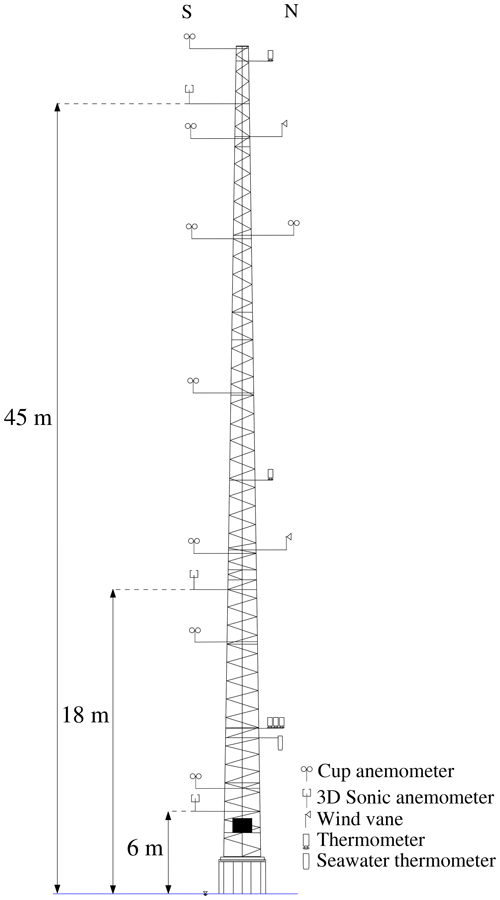Figure 3Sketch of the atmospheric instrumentation at SMW. The sonic anemometers are located in the southern boom “S” oriented at 226 from the north.

3 Theoretical background

## 3.1 Monin–Obukhov theory

The along-wind, cross-wind, and vertical velocity components are denoted as u, v, and w, respectively. Each component is split into a mean ($\stackrel{\mathrm{‾}}{u}$, $\stackrel{\mathrm{‾}}{v}$, $\stackrel{\mathrm{‾}}{w}$) and fluctuating part (u, v, w). In flat and homogeneous terrain, the flow is fairly horizontal; i.e. $\stackrel{\mathrm{‾}}{v}$ and $\stackrel{\mathrm{‾}}{w}$ are approximatively zero. Here, the fluctuating components are assumed to be stationary, Gaussian, ergodic random processes (Monin1958).

Although the u component drives the wind turbine's rotor fatigue loads, proper modelling of the v component in terms of power spectral density (PSD) and root coherence may be necessary for skewed flow conditions, which can occur because of a large wind direction shear or wind turbine yaw error . To estimate a wind turbine's fatigue loads, the vertical velocity component is likely more relevant in complex terrain than offshore . Nevertheless, this component is studied here for the sake of completeness. Also, the vertical velocity component provides precious information on the sonic anemometer flow distortion . The vertical velocity component is also necessary to assess the atmospheric stability using the eddy covariance method and facilitates the study of the waves' influences on the velocity data recorded by the sonic anemometers (e.g. Benilov et al.1974).

In the atmospheric surface layer, where Monin–Obukhov similarity theory (MOST) generally applies, the scaling velocity is the friction velocity u*, whereas the scaling lengths are the height z above the surface and the Obukhov length L , defined as

$\begin{array}{}\text{(1)}& L=-\frac{{u}_{*}^{\mathrm{3}}{\stackrel{\mathrm{‾}}{\mathit{\theta }}}_{\mathrm{v}}}{g\mathit{\kappa }\left(\stackrel{\mathrm{‾}}{{w}^{\prime }{\mathit{\theta }}_{\mathrm{v}}^{\prime }}\right)},\end{array}$

where ${\stackrel{\mathrm{‾}}{\mathit{\theta }}}_{\mathrm{v}}$ is the mean virtual potential temperature, g=9.81 m s−2 is the gravitational acceleration, κ≈0.4 is the von Kármán constant (Högström1985), and $\stackrel{\mathrm{‾}}{{w}^{\prime }{\mathit{\theta }}_{\mathrm{v}}^{\prime }}$ is the vertical flux of virtual potential temperature. For a given height z above the surface, the non-dimensional stability parameter $\mathit{\zeta }=z/L$ is used herein to classify the thermal stratification of the atmosphere.

While ${\mathit{\theta }}_{\mathrm{v}}^{\prime }$ can be fairly well approximated by the fluctuating sonic temperature measurement , the mean value ${\stackrel{\mathrm{‾}}{\mathit{\theta }}}_{\mathrm{v}}$ could not be reliably obtained from the sonic anemometers deployed on SMW (Kurt Hansen, personal communication, 2020). Therefore, ${\stackrel{\mathrm{‾}}{\mathit{\theta }}}_{\mathrm{v}}$ was obtained using the absolute temperature recorded from the Risø P2039 PT 100 sensor at 10 m a.m.s.l., which was converted into the virtual potential temperature using the pressure data from LM and assuming an air relative humidity of 90 % near the sea surface (Stull1988). The air pressure data from LM are used due to the absence of air pressure data at SMW and SMS.

Since the covariance between the cross-wind and the vertical component may not be negligible in the MABL , the friction velocity u* is computed as suggested by ; that is

$\begin{array}{}\text{(2)}& {u}_{*}=\sqrt[\mathrm{4}]{{\stackrel{\mathrm{‾}}{{u}^{\prime }{w}^{\prime }}}^{\mathrm{2}}+{\stackrel{\mathrm{‾}}{{v}^{\prime }{w}^{\prime }}}^{\mathrm{2}}}.\end{array}$

A common approach to assess the applicability of MOST is to study the non-dimensional mean wind speed profile ϕm defined as

$\begin{array}{}\text{(3)}& {\mathit{\varphi }}_{\mathrm{m}}\left(\frac{z}{L}\right)=\frac{\mathit{\kappa }z}{{u}_{*}}\frac{\partial \stackrel{\mathrm{‾}}{u}}{\partial z}\end{array}$

as a function of the atmospheric stability . In the following, ϕm is empirically modelled as by :

The validity of Eq. (4) is assessed for each anemometer in Sect. 5.1. It should be noted that the presence of waves, especially swell, may invalidate MOST in the first few metres above the surface , and this possibility will be discussed in Sect. 5.2. Under convective conditions, the validity of MOST may also be questionable if the fetch is only a few kilometres long due to the presence of internal boundary layers . In the present case, the choice of wind directions from 220 to 330 limits strongly the possibility that internal boundary layers affect the velocity measurements.

## 3.2 One-point turbulence spectrum

Appropriate modelling of the one-point velocity spectrum is required to compute reliably the dynamic wind-induced response and the power production of wind turbines . Integral turbulence characteristics, especially the turbulence intensity, are not always appropriate for turbulence characterisation , which motivates the study of the spectral characteristics of turbulence herein.

Following , the normalised surface-layer one-point velocity spectra express a universal behaviour in the inertial subrange:

$\begin{array}{}\text{(5)}& \frac{f{S}_{\mathrm{u}}\left(f\right)}{{u}_{*}^{\mathrm{2}}{\mathit{\varphi }}_{\mathit{ϵ}}^{\mathrm{2}/\mathrm{3}}}& \simeq \mathrm{0.3}{f}_{\mathrm{r}}^{-\mathrm{2}/\mathrm{3}}\phantom{\rule{0.25em}{0ex}}\mathrm{at}\phantom{\rule{0.25em}{0ex}}{f}_{\mathrm{r}}\gg \mathrm{1},\text{(6)}& \frac{f{S}_{\mathrm{v}}\left(f\right)}{{u}_{*}^{\mathrm{2}}{\mathit{\varphi }}_{\mathit{ϵ}}^{\mathrm{2}/\mathrm{3}}}& \approx \frac{f{S}_{\mathrm{w}}\left(f\right)}{{u}_{*}^{\mathrm{2}}{\mathit{\varphi }}_{\mathit{ϵ}}^{\mathrm{2}/\mathrm{3}}}\simeq \mathrm{0.4}{f}_{\mathrm{r}}^{-\mathrm{2}/\mathrm{3}}\phantom{\rule{0.25em}{0ex}}\mathrm{at}\phantom{\rule{0.25em}{0ex}}{f}_{\mathrm{r}}\gg \mathrm{1},\end{array}$

where ${f}_{\mathrm{r}}=fz/\stackrel{\mathrm{‾}}{u}$ and f is the frequency; Su, Sv, and Sw are the velocity spectra for the along-wind, cross-wind, and vertical velocity components, respectively; and ϕϵ is the non-dimensional turbulent kinetic energy dissipation rate . The latter is given by

$\begin{array}{}\text{(7)}& {\mathit{\varphi }}_{\mathit{ϵ}}=\frac{\mathit{\kappa }z\mathit{ϵ}}{{u}_{*}^{\mathrm{3}}},\end{array}$

where ϵ is the turbulent kinetic energy dissipation rate.

In the present case, ϕϵ is modelled as

## 3.3 The coherence of turbulence

The coherence of turbulence describes the spatial correlation of eddies. The root coherence is defined as the normalised cross-spectral density of turbulence and is a complex-valued function. The real part of the root coherence, known as the co-coherence, is one of the governing parameters for the structural design of wind turbines . At vertical separations, the co-coherence γi, where $i=\mathit{\left\{}u,v,w\mathit{\right\}}$, is defined as

$\begin{array}{}\text{(9)}& {\mathit{\gamma }}_{i}\left({z}_{\mathrm{1}},{z}_{\mathrm{2}},f\right)=\frac{Re\left\{{S}_{i}\left({z}_{\mathrm{1}},{z}_{\mathrm{2}},f\right)\right\}}{\sqrt{{S}_{i}\left({z}_{\mathrm{1}},f\right){S}_{i}\left({z}_{\mathrm{2}},f\right)}},\end{array}$

where ${S}_{i}\left({z}_{\mathrm{1}},{z}_{\mathrm{2}},f\right)$ is the two-point cross-spectral density between heights z1 and z2, whereas Si(z1,f) and Si(z2,f) are the one-point spectra estimated at heights z1 and z2, respectively.

proposed an empirical model to describe the co-coherence for vertical separations, which depends only on a decay parameter ci and a reduced frequency n:

$\begin{array}{}\text{(10)}& & {\mathit{\gamma }}_{i}\left(n\right)\approx \mathrm{exp}\left(-{c}^{i}n\right),\text{(11)}& & n=\frac{\mathrm{2}f{d}_{z}}{\stackrel{\mathrm{‾}}{u}\left({z}_{\mathrm{1}}\right)+\stackrel{\mathrm{‾}}{u}\left({z}_{\mathrm{2}}\right)},\end{array}$

where ${d}_{z}=\left|{z}_{\mathrm{1}}-{z}_{\mathrm{2}}\right|$. For three heights ${z}_{\mathrm{1}}>{z}_{\mathrm{2}}>{z}_{\mathrm{3}}$ such that ${z}_{\mathrm{1}}-{z}_{\mathrm{2}}={z}_{\mathrm{2}}-{z}_{\mathrm{3}}$, Davenport's model predicts that ${\mathit{\gamma }}_{i}\left({z}_{\mathrm{1}},{z}_{\mathrm{2}},f\right)$ and ${\mathit{\gamma }}_{i}\left({z}_{\mathrm{2}},{z}_{\mathrm{3}},f\right)$ collapse onto a single curve when expressed as a function of n. This behaviour, referred to as Davenport's similarity herein, is questioned by for vertical separations and by and for lateral separations.

modified the Davenport model by assuming that ci was a linear function of the distance; i.e.

$\begin{array}{}\text{(12)}& {c}^{i}={c}_{\mathrm{1}}^{i}+\frac{\mathrm{2}{c}_{\mathrm{2}}^{i}{d}_{z}}{\left({z}_{\mathrm{1}}+{z}_{\mathrm{2}}\right)},\end{array}$

where ${c}_{\mathrm{1}}^{i}$ and ${c}_{\mathrm{2}}^{i}$ are constants. Equation (12) reflects the blocking by the ground or the sea surface, which leads to an increase in the co-coherence with measurement height. This equation implies that the co-coherence decreases more slowly than predicted by the Davenport model if measurements are conducted far from the surface and at short separations. On the other hand, the co-coherence may decrease faster than predicted by the Davenport model if the measurements are associated with large separation distances. This implies that fitting the Davenport model to measurements with short or large separations may lead to an inadequate design of wind turbines.

The model by was further modified by by including a third decay parameter ${c}_{\mathrm{3}}^{i}$ to account for the fact that the co-coherence cannot reach values of 1 at zero frequency unless the separation distance is zero. This led to the following three-parameter co-coherence function, which is herein referred to as the modified Bowen model:

$\begin{array}{}\text{(13)}& & {\mathit{\gamma }}_{ii}\left({z}_{\mathrm{1}},{z}_{\mathrm{2}},f\right)=\mathrm{exp}\left(-{f}_{a}\right)\mathrm{exp}\left(-{f}_{b}\right),\text{(14)}& & {f}_{a}=\frac{\left|{z}_{\mathrm{2}}-{z}_{\mathrm{1}}\right|}{\stackrel{\mathrm{‾}}{u}\left({z}_{\mathrm{1}},{z}_{\mathrm{2}}\right)}\sqrt{{\left({c}_{\mathrm{1}}^{i}f\right)}^{\mathrm{2}}+{\left({c}_{\mathrm{3}}^{i}\right)}^{\mathrm{2}}},\text{(15)}& & {f}_{b}=\frac{\mathrm{2}{c}_{\mathrm{2}}^{i}f{\left|{z}_{\mathrm{2}}-{z}_{\mathrm{1}}\right|}^{\mathrm{2}}}{\left({z}_{\mathrm{1}}+{z}_{\mathrm{2}}\right)\stackrel{\mathrm{‾}}{u}\left({z}_{\mathrm{1}},{z}_{\mathrm{2}}\right)}.\end{array}$

It should be noted that both ${c}_{\mathrm{1}}^{i}$ and ${c}_{\mathrm{2}}^{i}$ are dimensionless, whereas ${c}_{\mathrm{3}}^{i}$ has the dimension of the inverse of a time. Following , ${c}_{\mathrm{3}}^{i}\propto \mathrm{1}/T$, where T is a timescale of turbulence. Therefore, low values of ${c}_{\mathrm{3}}^{i}$ are associated with a co-coherence converging toward 1 at low frequencies for which the separation distance is small compared to a typical turbulence length scale. The rotor diameter of multi-megawatt OWTs commissioned after 2015 in the North Sea is slightly larger than 150 m. For such structures, assuming ${c}_{\mathrm{3}}^{i}\approx \mathrm{0}$ may no longer be appropriate.

recommends the use of two empirical coherence formulations. The first one was derived based on the exponential coherence proposed by , which read as

$\begin{array}{}\text{(16)}& {\mathit{\gamma }}_{\mathrm{u}}\left(f,{d}_{z}\right)=\mathrm{exp}\left\{-\mathrm{12}\left[\sqrt{{\left(\frac{f{d}_{z}}{{\stackrel{\mathrm{‾}}{u}}_{\mathrm{hub}}}\right)}^{\mathrm{2}}+{\left(\mathrm{0.12}\frac{{d}_{z}}{\mathrm{8.1}{L}_{\mathrm{c}}}\right)}^{\mathrm{2}}}\right]\right\},\end{array}$

where ${\stackrel{\mathrm{‾}}{u}}_{\mathrm{hub}}$ is the mean wind speed at the hub height and

The second coherence model was derived based on a spectral tensor of homogeneous turbulence (Mann1994) but is not described in detail here. Further assessments of this model can be found in, for example, Mann (1994), , and .

4 Data processing

Sonic anemometer data monitored continuously from May 1994 to July 1995 were selected. No data were collected in July and October 1994, leading to 13 months of available records. The sonic anemometer at z=18 m was chosen as the reference sensor throughout the data processing. The measurements at z=45 m were associated with higher measurement noise than at the other two heights. Although this noise was almost negligible at wind speeds above 10 m s−1, it was visible in the velocity records at low wind speeds.

Since the wind sensors at 6, 18, and 45 m a.m.s.l. were omnidirectional sonic anemometers, they were prone to flow distortion by the transducer. This flow distortion was investigated in terms of friction velocity estimated from the asymmetric solent anemometer mounted at 10 m a.m.s.l., between May and September 1994 only due to data availability. The corrected friction velocities for the sensors at 6, 18, and 45 m were computed using the data at 10 m, as elaborated in Appendix B. When using the corrected friction velocity, no significant improvement was found for the ensemble-averaged normalised PSD estimates. It was then concluded that for the relatively narrow selected sector (220–330), the application of an ensemble averaging limits the influence of the transducer-induced flow distortion on the spectral flow characteristics. Therefore, it was decided not to apply a correction for both friction velocity and the Obukhov length to avoid over-processing the data.

Both the double-rotation technique and the sectoral planar fit (PF) method were considered to correct the tilt angles of the SAs. The choice of the algorithm relied on a comparison between the friction velocity u* estimated using Eq. (2) and the method by , which does not require any tilt correction. The latter method provides an estimate u*R of the friction velocity using the eigenvalues of the Reynolds stress tensor. Following this comparison, the double-rotation technique was found to provide, in the present case, slightly more reliable results than the PF algorithm (see Appendix A). It should be noted that this finding is likely specific to the Vindeby dataset as the planar fit method usually provides better estimates of the turbulent fluxes .

The time series were sometimes affected by outliers. Here, the outliers were identified using a moving median window based on a 5 min window length. The same outlier detection algorithm was also used for the sea surface elevation data but with a moving window of 180 s. The local median values were then used to compute the median absolute deviation (MAD), as recommended by . Data located more than five MADs away from the median were replaced with NaNs (NaN denotes “not a number”). The generalised extreme Studentised deviate test (Rosner1983) was also assessed to detect outliers but did not bring significant improvements. When the number of NaNs in the time series was under 5 %, the NaNs were replaced using a non-linear interpolation scheme based on the inpainting algorithm by with the “spring” method. A more adequate but slower approach using autoregressive modelling (Akaike1969) was also applied but yielded a similar conclusion and therefore was not used. Time series containing more than 5 % of NaNs were dismissed. Although other spike detection and interpolation algorithms exist in the literature (e.g. ), the approach adopted in this study was found to provide an adequate trade-off between computation time and accuracy.

To assess the first- and second-order stationarity of the velocity recordings, the moving mean and the moving standard deviation of the along-wind component were calculated using a window length of 10 min. The time series were considered stationary when the two following criteria were fulfilled: (1) the maximum absolute relative difference between the moving mean and the static mean was lower than a threshold value of 20 %; (2) for the moving standard deviation, the maximum absolute relative difference was also used with a threshold value of 40 %. The choice of a larger threshold value for the moving standard deviation test is justified by the larger statistical uncertainty associated with the variance of a random process compared to its mean .

Velocity records with an absolute value of skewness larger than 2 or a kurtosis below 1 or above 8 are likely to display an unphysical behaviour and were subsequently dismissed. The statistical uncertainties in the records were quantified as by and :

$\begin{array}{}\text{(18)}& & {a}_{ii}^{\mathrm{2}}=\frac{\mathrm{4}z}{T\stackrel{\mathrm{‾}}{u}}\left[\frac{\stackrel{\mathrm{‾}}{{{i}^{\prime }}^{\mathrm{4}}}}{{\mathit{\sigma }}_{i}^{\mathrm{4}}}-\mathrm{1}\right],\text{(19)}& & {a}_{\mathrm{uw}}^{\mathrm{2}}=\frac{z}{T\stackrel{\mathrm{‾}}{u}}\left[\frac{\stackrel{\mathrm{‾}}{\left({u}^{\prime }{w}^{\prime }{\right)}^{\mathrm{2}}}}{{u}_{*}^{\mathrm{4}}}-\mathrm{1}\right],\end{array}$

$\begin{array}{}\text{(20)}& {a}_{\mathrm{vw}}^{\mathrm{2}}=\frac{z}{T\stackrel{\mathrm{‾}}{u}}\left[\frac{\stackrel{\mathrm{‾}}{\left({v}^{\prime }{w}^{\prime }{\right)}^{\mathrm{2}}}}{{u}_{*}^{\mathrm{4}}}-\mathrm{1}\right],\end{array}$

where aij with $i,j=\left(u,v,w\right)$ is the uncertainty associated with the variance and covariance estimates. Time series with a large random error, i.e. aii>0.20 or aij>0.50 with ij, were excluded.

The records with a mean wind speed below 5.0 m s−1 at 18 m a.m.s.l. were discarded. Assuming a logarithmic mean wind profile, a near-neutral atmosphere, and a roughness length z0 of 0.0002 m (WMO2008), the corresponding mean wind speed at a typical offshore wind turbine hub height (90 m a.m.s.l.) is 5.7 m s−1. The present choice of a lower mean wind speed threshold is, therefore, consistent with the cut-in wind speed of large offshore wind turbines, which is 5.0 m s−1 at hub height. It also ensures a consistent comparison of the spectral characteristics of turbulence with the data collected at FINO1, where the lowest mean wind speed considered was 5.0 m s−1 at 80 m a.m.s.l.

The PSD estimates of the velocity fluctuations were evaluated using Welch's method (Welch1967) with a Hamming window, three segments, and 50 % overlap. The spectra were ensemble-averaged using the median of multiple 30 min time series that passed the data-quality tests described above and were smoothed by using bin averaging over logarithmically spaced bins. The co-coherence estimates were also computed using Welch's method but using eight segments and 50 % overlap to further reduce the statistical uncertainty.

Table 1 displays the percentage of samples at each measurement height that failed the data-quality assessment. It relies on initial data availability of 86 %, 97 %, and 86 % for the anemometers at 6, 18, and 45 m a.m.s.l., respectively. Following the criteria used in the data processing and Table 1, the percentages of data considered for the analysis were 69 %, 76 %, and 45 % at 6, 18, and 45 m a.m.s.l., respectively. These percentages correspond to 1566 time series of 30 min duration for the SA at 6 m, 1771 time series at 18 m, and 854 at 45 m. The data from SA at 45 m showed the highest portion of non-stationary and large statistical uncertainties compared to the other SAs. Furthermore, the SA at 45 m also contained the highest fraction of NaNs in the time series, due to a large number of outliers. The larger fraction of data removal for the anemometer at 45 m is attributed to the observed uncorrelated white noises in the signal. This measurement noise, which may be linked to the length of the cable joining the anemometer and the acquisition system, is usually small for wind speed above 10 m s−1. Therefore, it was decided not to filter it out using digital low-pass filtering techniques. Time series that were flagged as non-physical made up less than 5 % for each SA in the present dataset, likely because the test was applied after the outlier detection algorithm. The portion of non-stationary time series increased with height (see Table 1). Closer to the surface, the eddies are smaller and are less likely to be affected by the sub-mesospheric and mesospheric atmospheric motion, which contributes to non-stationary fluctuations .

Table 1Percentage of the records between April 1994 and July 1995 from SMW that failed the data-quality assessment.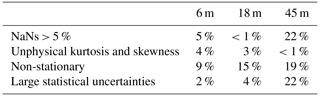5 Results

## 5.1 Applicability of MOST

In the atmospheric surface layer, the friction velocity u* is often assumed constant with the height (constant flux layer). However, Fig. 4 shows that the friction velocity is generally larger at 6 m than at the other two measurement heights, especially under stable conditions. The different friction velocity values at 6 m compared to 18 and 45 m were suspected to be due to the transducer-induced flow distortion and/or the contribution of the wave-induced stress to the total turbulent stress .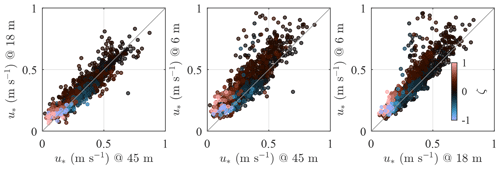Figure 4Friction velocity estimated by the three sonic anemometers on SMW for a wide range of stability conditions with $|\mathit{\zeta }|<\mathrm{2}$.

The applicability of MOST is assessed by studying ϕm as a function of ζ. The similarity relation describing the mean wind speed profile agrees well with the sonic anemometer measurements under all stability conditions except between the sensor at 6 m and 18 m a.m.s.l. at ζ>0.3. It should be noted that at 6 m a.m.s.l., the local estimate of ζ shows a much greater portion of near-neutral conditions than at 18 m a.m.s.l. The right panel of Fig. 5 does not show such a deviation, maybe because the friction velocity estimated at 45 m a.m.s.l. is slightly underestimated due to the high-frequency noise in the velocity records of the top sensor. This further justifies the use of the sonic anemometer at 18 m a.m.s.l. to estimate the non-dimensional stability parameter ζ.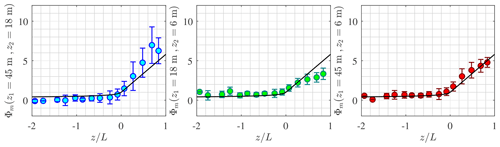Figure 5Variation in ϕm with the non-dimensional stability parameter ζ estimated from SA at 18 m a.m.s.l. The solid black line is Eq. (4), and the error bar represents the interquartile range.

The distribution of ζ as a function of the mean wind speed $\stackrel{\mathrm{‾}}{u}$ is given in Fig. 6 for the sector between 220 and 330. The majority (82 %) of the stationary record samples were associated with a wind speed between 7–15 m s−1 at 18 m a.m.s.l. Non-neutral conditions are defined herein as situations where $|\mathit{\zeta }|>\mathrm{0.1}$. They represent 69 % of the samples at $\stackrel{\mathrm{‾}}{u}<\mathrm{12}$ m s−1 and 12 % at $\stackrel{\mathrm{‾}}{u}\ge \mathrm{12}$ m s−1. The distribution of the atmospheric stability conditions is in overall agreement with and for the Vindeby site.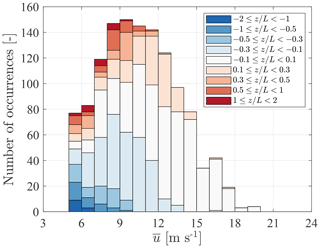Figure 6Stability distribution as a function of mean wind speed for the considered fetches (220–330) at height z=18 m.

Strongly unstable or stable stratifications ($|\mathit{\zeta }|>\mathrm{0.5}$) made up only about 8 % of the total number of samples (Fig. 6). A low number of samples can lead to large uncertainties when comparing the flow characteristics between SMW and FINO1 for specific stability bins. Therefore, only stability conditions satisfying $|\mathit{\zeta }|\le \mathrm{0.5}$ are discussed herein unless indicated otherwise.

## 5.2 Wind–wave interactions

The fairly close proximity of the sensor at 6 m to the sea surface is used to study the potential influence of the wave boundary layer (WBL) . This layer is also called the wave sublayer by , who suggest that its depth is approximately 5Hs, although there is no consensus on this value. The objective of this subsection is to identify whether the wave-induced turbulence can be detected in the velocity records at 6 m a.m.s.l.

The wave elevation data collected by the AWR near SMW are explored herein in terms of wind–wave interactions. A total of 925 high-quality samples collocated in time with the wind velocity data were identified. Each wave elevation record was 30 min long and corresponded to a wind direction between 220 and 330. There exist methods to filter out the wave-induced velocity component from the turbulent velocity component (e.g. ), but these methods are not addressed herein for brevity.

The interactions between wind turbulence and the sea surface were explored in terms of the co-coherence and the quad-coherence (the imaginary part of the root coherence) between the vertical velocity component w and the velocity of the wave surface $\stackrel{\mathrm{˙}}{\mathit{\eta }}=\mathrm{d}\mathit{\eta }/\mathrm{d}t$. Similar approaches were adopted earlier by, for example,  or but using the coherence and without taking advantage of the ensemble average to reduce the systematic and random error, which are typically associated with the root-coherence function. In the present case, neither the co-coherence nor the quad-coherence between $\stackrel{\mathrm{˙}}{\mathit{\eta }}$ and w differs significantly from zero for Hs<0.7 m. For the sensor at 6 m a.m.s.l., a non-zero root coherence was discernible from the background noise at 0.7 m <Hs< 0.9 m. The co-coherence and quad-coherence estimates were significantly different from zero for Hs>0.9 m, as illustrated in Fig. 7, where the ensemble averaging of the 60 samples was applied to reduce the random error. The inset in Fig. 7 shows that the selected records are characterised by a single spectral peak fp located at frequencies between 0.20 and 0.25 Hz, which is the frequency range where the quad-coherence is substantially different from zero. The observed co-coherence and the quad-coherence at this frequency range show the 90 out-of-phase fluctuations between $\stackrel{\mathrm{˙}}{\mathit{\eta }}$ and w, where the latter is lagging. The co-coherence and quad-coherence estimates between $\stackrel{\mathrm{˙}}{\mathit{\eta }}$ and the horizontal wind component u were also investigated but were nearly zero for the three sonic anemometers on SMW.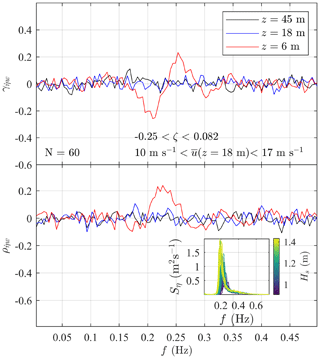Figure 7Co-coherence ${\mathit{\gamma }}_{\stackrel{\mathrm{˙}}{\mathit{\eta }}\mathrm{w}}$ and quad-coherence ${\mathit{\rho }}_{\stackrel{\mathrm{˙}}{\mathit{\eta }}\mathrm{w}}$ between the velocity of the wave surface $\stackrel{\mathrm{˙}}{\mathit{\eta }}$ and the vertical wind velocity w from the three sonic anemometers on SMW. The inset shows the individual wave elevation spectra Sη associated with Hs>0.9 m (60 samples) used to estimate ${\mathit{\gamma }}_{\stackrel{\mathrm{˙}}{\mathit{\eta }}\mathrm{w}}$ and ${\mathit{\rho }}_{\stackrel{\mathrm{˙}}{\mathit{\eta }}\mathrm{w}}$.

The limited number of data showing a clear correlation between the velocity of the sea surface and the vertical wind component implies that the wave-induced turbulence has a limited impact on the anemometer records at 6 m. The influence of the sea surface elevation on the vertical turbulence was not clearly visible in the one-point vertical velocity spectra Sw, except for at Hs>1.2 m, where a weak spectral peak near 0.2 Hz was distinguishable. The wave-induced fluctuating wind component is generally much weaker compared to the wind turbulence as highlighted by, for example, , and . An exception may be the case of weak wind and swell conditions, which are more likely to result in the observation of a sharp spectral peak near fp in the Sw spectrum . Nonetheless, as previously mentioned, such conditions are rare near SMW, most likely because SMW was located in a relatively sheltered closed-water environment rather than in the open ocean.

## 5.3 Turbulence spectra

When performing numerical simulations to compute the wind-induced response of wind turbines, an essential input to model the wind inflow conditions is the PSD of the velocity fluctuations. Figures 810 depict the PSD estimates for the along-wind, cross-wind, and vertical wind components, respectively, as a function of the reduced frequency fr for five stability classes. Surface-layer scaling is adopted; i.e. the PSDs are normalised with u* (Eq. 2) and ${\mathit{\varphi }}_{\mathit{ϵ}}^{\mathrm{2}/\mathrm{3}}$ (Eq. 8). The number of available samples for each stability class is denoted as N and displayed in each panel.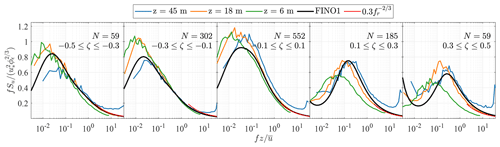Figure 8Normalised spectra of the along-wind component at 45, 18, and 6 m a.m.s.l. for various stability conditions. The red curve is derived from Eq. (5), and N denotes the number of samples considered for ensemble averaging.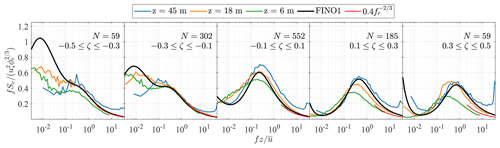Figure 9Normalised spectra of the cross-wind component at 45, 18, and 6 m a.m.s.l. for various stability conditions. The red curve is derived from Eq. (6), and N denotes the number of samples considered for ensemble averaging.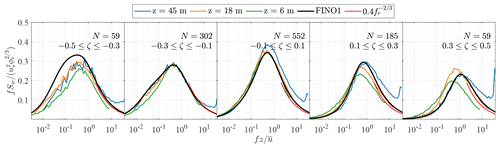Figure 10Normalised spectra of the vertical wind component at 45, 18, and 6 m a.m.s.l. for various stability conditions. The red curve is derived from Eq. (6), and N denotes the number of samples considered for ensemble averaging.

Figures 8 to 10 compare the estimated spectra at z=45 m, z=18 m, and z=6 m a.m.s.l. with the empirical model established on FINO1 (solid black line) at z=41 m a.m.s.l. . The red curves represent the high-frequency asymptotic behaviour of surface-layer spectra for each stability class. It should be noted that the latter curves do not indicate when the inertial subrange starts since the frequencies they cover were arbitrarily chosen.

In Fig. 8, the maximum values of the normalised spectra for near-neutral conditions ($-\mathrm{0.1}\le \mathit{\zeta }\le \mathrm{0.1}$) are close to unity, as described by . The velocity spectra estimated at 45 m a.m.s.l. sometimes show deviations from the surface-layer scaling under near-neutral and stable conditions, likely due to the observed aforementioned uncorrelated high-frequency noise, which leads to an underestimation of the friction velocity. Under light and moderate unstable conditions, i.e. $-\mathrm{0.3}\le \mathit{\zeta }\le -\mathrm{0.1}$, the velocity spectra at 6 m and 18 m a.m.s.l. are similar, which supports the idea that the wave sublayer is shallower than 6 m. As mentioned in Sect. 5.1, the non-dimensional stability parameter ζ estimated at 6 m reflected the predominance of near-neutral conditions. This results in discrepancies between the spectral estimates at 6 and 18 m in Figs. 8 to 10 which increase with $|\mathit{\zeta }|$.

Following surface-layer scaling, the normalised spectra at different heights should collapse onto one single curve at high frequencies, which was observed at heights between 40 and 80 m a.m.s.l., at FINO1 for $|\mathit{\zeta }|<\mathrm{1}$. However, this is not always the case in Figs. 810. Deviations from surface-layer scaling may be partly attributed to transducer-induced flow distortion. Regarding the velocity data at 45 m a.m.s.l., the measurement noises lift the high-frequency range of the velocity spectra above the spectral slope predicted by Eq. (5) or Eq. (6). At 18 m a.m.s.l., Eqs. (5) and (6) predict remarkably well the velocity spectra at fr>3, indicating that surface-layer scaling is applicable at this height.

The presence of the spectral gap , separating the microscale fluctuations from the sub-mesoscale and mesoscale ones, is noticeable at ζ>0.3, in line with previous observations . Under stable conditions, the spectral gap seems to move toward lower frequencies as the height above the surface decreases. This contrasts with the observations from an onshore mast on Østerild (Denmark) by , which indicated that the location of the spectral gap on the frequency axis was relatively constant with height.

As mentioned by , the spectral gap timescale can be only a few minutes long under stable conditions. For ζ>0.3, the averaging period selected in the present study may be too large to provide reliable integral turbulence characteristics. However, filtering out the mesoscale motion may not be desirable for structural design purposes since operating wind turbines experience both turbulence and mesoscale fluctuations . In this regard, the use of spectral flow characteristics to parametrise the wind loading on OWTs is preferable.

Under near-neutral conditions, the sensors at 6 and 18 m a.m.s.l. are likely located in the so-called eddy surface layer , where the sea surface blocks the flow and distorts eddies. This leads to a flat spectral peak. As a result, the integral length scale would be estimated with large uncertainties. Such a spectral behaviour has also been observed above the eddy surface layer , but its consequences for wind turbine loads are unclear.

Overall, the velocity spectra estimated at 18 and 45 m a.m.s.l. at Vindeby match well with the empirical spectra estimated at 41 m a.m.s.l. on FINO1 for $-\mathrm{0.5}\le \mathit{\zeta }<\mathrm{0.5}$. This comparison is encouraging for further explorations of the surface-layer turbulence characteristics at coastal and offshore sites. Nonetheless, detailed wind measurements at heights of z≥100 m are needed to obtain a complete overview of the turbulence characteristics in the MABL that is relevant for OWT designs.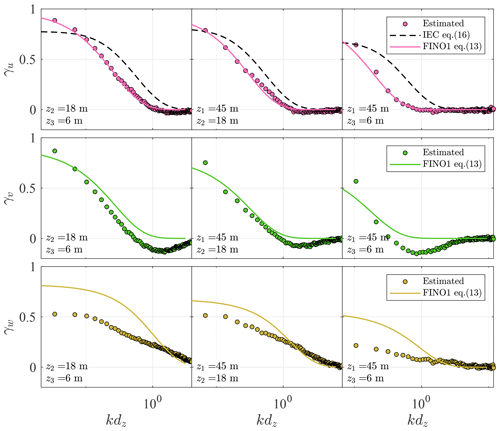Figure 11Co-coherences of the along-wind (top panels), lateral (middle panels), and vertical velocity components (lower panels) for $|\mathit{\zeta }|\le \mathrm{0.1}$ at three different vertical separation distances. The dots represent the measurement, and the lines mark the predictions using the IEC exponential coherence model (dashed line) and the modified Bowen model (solid line) with the fitted coefficients from FINO1 (Cheynet2019).

## 5.4 Co-coherence of turbulence

The vertical co-coherence of the along-wind, cross-wind, and vertical wind components are denoted by γu, γv, and γw, respectively. Under near-neutral conditions ($|\mathit{\zeta }|\le \mathrm{0.1}$), these are expressed as a function of kdz in Fig. 11 where $k=\mathrm{2}\mathit{\pi }f/\stackrel{\mathrm{‾}}{u}$ is the wave number, assuming that turbulence is frozen (Taylor1938). The co-coherence estimates are presented for three separation distances dz because three measurement heights (z1=45 m, z2=18 m, and z3=6 m) were used. The co-coherences estimated on SMW are compared to the IEC coherence model (Eq. 16) and the modified Bowen model (Eq. 13). For the latter model, the parameters estimated on FINO1 (Cheynet2019) are directly used. The decay coefficients used for Eq. (13) were, therefore, $\left[{c}_{\mathrm{1}}^{\mathrm{u}},{c}_{\mathrm{2}}^{\mathrm{u}},{c}_{\mathrm{3}}^{\mathrm{u}}\right]=\left[\mathrm{6.0},\mathrm{17.8},\mathrm{0.02}\right]$ and $\left[{c}_{\mathrm{1}}^{\mathrm{w}},{c}_{\mathrm{2}}^{\mathrm{w}},{c}_{\mathrm{3}}^{\mathrm{w}}\right]=\left[\mathrm{2.7},\mathrm{4.0},\mathrm{0.16}\right]$ as well as $\left[{c}_{\mathrm{1}}^{\mathrm{v}},{c}_{\mathrm{2}}^{\mathrm{v}},{c}_{\mathrm{3}}^{\mathrm{v}}\right]=\left[\mathrm{0},\mathrm{23.0},\mathrm{0.09}\right]$.

Figure 11 shows that the coefficients of the modified Bowen model estimated on FINO1 apply very well to γu estimated on SMW. Larger deviations are observed for the cross-wind components, for which γv displays large negative values, especially for separations between 6 and 45 m a.m.s.l. At FINO1, the negative part of γv was relatively small, which justified the use of Eq. (13) with no negative co-coherence values. Following , , or , the negative part is a consequence of the phase difference and is non-negligible for the cross-wind component, which is also observed in the present case. Since this phase difference increases with the mean wind shear, it is more visible at SMW than at FINO1, where the measurements are at greater heights than at SMW. The co-coherence of the vertical component estimated on SMW deviates from the one fitted to observations at the FINO platform. The source of such deviations remains unclear.

The IEC exponential coherence model over-predicts γu when the measurement height decreases and when the separation distance increases because this model follows fairly well Davenport's similarity, except at kdz<0.1. In , the Davenport model was suspected to lead to an overestimation of the turbulent wind loading on OWTs. The present results indicate that a similar overestimation may be obtained if the IEC exponential coherence model is used. Further studies are, however, needed to better quantify this possible overestimation in terms of dynamic wind loading on the wind turbine's rotor and tower, as well as on the floater's motions in the case of a floating wind turbine. Finally, additional data collection is needed to study the co-coherence at lateral separations, which is required for wind turbine design since it was not available at FINO1 or SMW.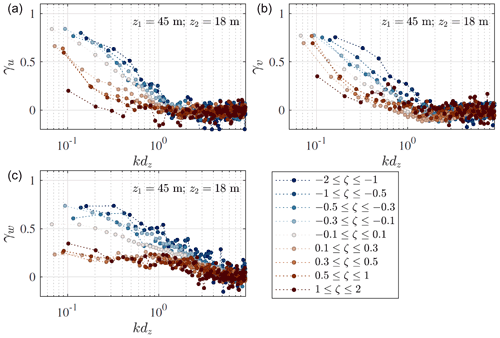Figure 12Co-coherence of the along-wind (a), cross-wind (b), and vertical (c) velocity components for nine stability classes, given by $\mathit{\zeta }=z/L$. The separation distance is 27 m between the sensors at 18 m and 45 m a.m.s.l.

To demonstrate the effect of the thermal stratification on the observed co-coherence at SMW, Fig. 12 shows the estimated co-coherence for $-\mathrm{2}\le \mathit{\zeta }\le \mathrm{2}$ for the three turbulence components. As observed by, for example,  or and modelled by , the vertical co-coherence is generally highest for convective conditions and smallest for stable conditions. Such results reinforce the idea that modelling the turbulent loading on offshore wind turbines using a coherence model established for neutral conditions may only be appropriate for the ultimate limit state design but not for the fatigue life design.

## 5.5 Relevancy of the database for load calculation of OWTs

This study provides a thorough overview of the MABL spectral turbulence characteristics with respect to the variation in the atmospheric stability at SMW. However, its direct applicability for the designs of OWTs should be assessed carefully due to the assumptions made in the data analysis.

The presented results do not include the non-stationary conditions encountered in the field, which were removed before the analysis. About 20 % of the data were disregarded as non-stationary to establish reliable spectra and co-coherence estimates. In the present case, non-stationary fluctuations were mainly associated with frequencies close to or below 0.05 Hz. For typical spar-type and semisubmersible OWT floaters, these frequencies encompass the quasi-static motions and a few of the lowest eigenfrequencies of the floaters . Additionally, the non-stationary turbulence fluctuations could result in non-Gaussian loadings, which could further lead to underestimation of fatigue loading .

Furthermore, the present dataset was recorded at heights lower than the hub height of the recent and the future OWTs, which is around 130 m (e.g. GE Renewable Energy2021). At such heights, MOST may no longer be applicable . Above the surface layer, the velocity spectra may become independent of the height above the surface, which is coarsely accounted for in .

6 Conclusions

This study explores the turbulence spectral characteristics from wind records of a year duration on an offshore mast called Sea Mast West (SMW) near the first offshore wind farm Vindeby. We aim to identify similarities between the turbulence characteristics estimated on the FINO1 platform in the North Sea and those at Vindeby. Such an investigation is crucial to establish appropriate turbulence models relevant for the design of offshore wind turbines (OWTs). The dataset analysed was acquired by 3D sonic anemometers at 6, 18, and 45 m a.m.s.l., which complements the dataset collected between 40 m and 80 m a.m.s.l. on FINO1 .

The correlation between the sea surface elevation and the vertical turbulent fluctuations at 6 m a.m.s.l. is quantified in terms of co-coherence between the vertical turbulent component and the velocity of the sea surface elevation. However, it is clearly visible for significant wave heights Hs exceeding 0.9 m only. Therefore, it is concluded that the sonic anemometers are located above the wave boundary layer most of the time.

The measurements at 18 m a.m.s.l. follow fairly well surface-layer scaling, as expected. Because the sensors at 6 and 18 m are located in the lower part of the surface layer, a wide spectral peak for near-neutral stratification is observed, which reflects the distortion of the eddies as they scrape along the surface. For $\mathit{\zeta }=|z/L|\le \mathrm{0.3}$, the power spectral density of the along-wind velocity component at 18 and 45 m is consistent with the empirically defined spectral models estimated at 41 m on FINO1 . In the present case, most of the wind records are associated with $|\mathit{\zeta }|<\mathrm{0.3}$. Nonetheless, for $|\mathit{\zeta }|>\mathrm{0.3}$, deviations from the empirical spectral model fitted to the data recorded on the FINO1 platform may be attributed to transducer-induced flow distortion and/or limited applicability of the surface-layer scaling.

The co-coherence estimates of the along-wind component for neutral atmospheres are well described by the same three-parameter exponential decay function as used at FINO1 (Cheynet2019). However, this is not the case for the lateral wind components due to the closer distance to the sea surface, which amplifies the phase differences between measurements at two different heights. For the vertical component, the co-coherence decreases faster than the predicted values at FINO1 . Under stable stratification, the co-coherence estimates of the three turbulent components (γu, γv, and γw) are significantly lower than for near-neutral conditions, in particular for kdz<1. On the other hand, γu, γv, and γw are slightly higher for convective conditions compared to near-neutral conditions at kdz<1. Since the co-coherence is one of the governing parameters for wind loading on structures, its dependency on the atmospheric stability, which is rarely documented in the marine atmospheric boundary layer, may become essential to establishing design criteria for OWT fatigue life. The variability in Hywind Scotland wind turbines’ floater motion with atmospheric stability may be one example that demonstrates the importance of stability-corrected co-coherence in OWT responses.

Although the Vindeby dataset is located below recent wind turbines' sizes, this does not mean that the dataset may not be useful for wind turbine design purposes. In fact, the widely known Kansas spectra were based on the observations at heights not exceeding 30 m and have been adopted in . From the Vindeby dataset, we found some similarities with the Kansas spectra for near-neutral conditions. For non-neutral conditions, the turbulence spectra at height 45 m a.m.s.l. have a consistent behaviour with the predicted spectra at FINO1 at 41.5 m a.m.s.l. The comparison between the turbulence characteristics at Vindeby and FINO1 is therefore valuable to further develop comprehensive spectral turbulence models that are suitable for modern OWT designs. Nevertheless, future atmospheric measurements at heights up to 250 m a.m.s.l. are necessary to obtain the knowledge of turbulence characteristics where the surface-layer scaling may no longer be applicable.

Appendix A: Sonic anemometer tilt correction

The friction velocity estimates using the double-rotation technique, sectoral planar fit, and the method by are compared in Fig. A1. In general, the friction velocity estimates from all methods are in good agreement. The average correlation coefficient for all heights is 0.985 for $|\mathit{\zeta }|\le \mathrm{2}$. The PF algorithm leads to a slightly larger scatter between u*R and u*, where the average correlation coefficient from all heights is 0.976 for $|\mathit{\zeta }|\le \mathrm{2}$ (Table A1). The double-rotation algorithm seems to give a smaller deviation between u*R and u* than the PF algorithm in the present study, which justified the adoption of the double rotation as a tilt correction method herein.

noted that u*R is appropriate to estimate the friction velocity if the thermal stratification of the atmosphere is neutral only. Yet, Fig. A1 suggests that Klipp's method performs well for non-neutral conditions too, as highlighted by the correlation coefficients in Table A1, which vary between 0.963 and 0.989. Additional studies using measurements from other coastal or offshore sites are needed to assess if such observations are recurring.

The angle between the stress vector and the wind vector is given as $\mathit{\alpha }=\mathrm{arctan}\left(\stackrel{\mathrm{‾}}{{v}^{\prime }{w}^{\prime }}/\stackrel{\mathrm{‾}}{{u}^{\prime }{w}^{\prime }}\right)$ . It is found that α increases from 8 at 6 m a.m.s.l. to 13 at 45 m a.m.s.l. when $\stackrel{\mathrm{‾}}{{v}^{\prime }{w}^{\prime }}<\mathrm{0}$. When $\stackrel{\mathrm{‾}}{{v}^{\prime }{w}^{\prime }}>\mathrm{0}$, α is almost constant with the height with an average value of −7. The relatively low value of α, therefore, suggests that the direction of the wind–wave-induced stress is fairly well aligned with the mean wind direction near SMW.

Table A1Correlation coefficients between u*R and u* using the planar fit (PF) or double rotation (DR).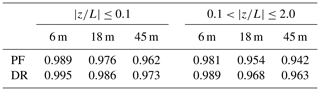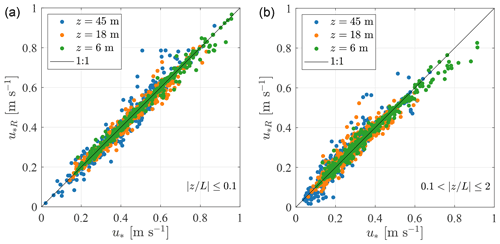Figure A1Friction velocity computed using the eddy covariance method with the double-rotation method compared with the Klipp method. Panel (a) considers only $|z/L|\le \mathrm{0.1}$, and panel (b) considers $\mathrm{0.1}<|z/L|\le \mathrm{2}$.

The sonic anemometers mounted at 6, 18, and 45 m a.m.s.l. were omnidirectional solent anemometers, which can be prone to flow distortion by the transducer. Between May and September 1994, a Gill solent anemometer with an asymmetric head was installed at 10 m a.m.s.l. on the southern boom of SMW (i.e. on the same side as the other three anemometers). The asymmetric head reduces the flow distortion by the transducer, at least for a specific wind sector. Although the flow distortion by the asymmetric solent was actually unknown, this sensor was used to assess the error in the friction velocity calculated with the omnidirectional solent anemometers. Only wind directions from 220 to 330 were selected as they corresponded to the sector investigated in the present study.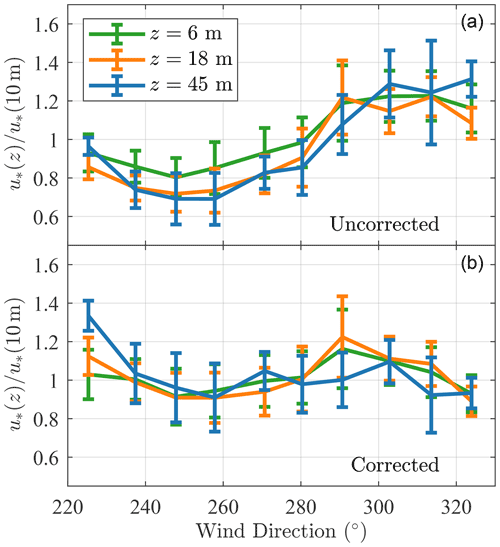Figure B1Ratio of the friction velocity by the omnidirectional solent anemometers to the one estimated at 10 m (asymmetric solent anemometer) before (a) and after (b) correction using a multivariate regression analysis. Velocity data recorded between May and September 1994 for the sector 220–330 were used (480 samples of 30 min duration), and $|z/L|<\mathrm{2}$ at 10 m a.m.s.l.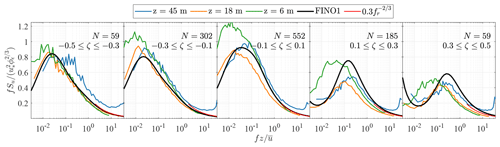Figure B2Normalised spectra of the along-wind component on SMW with the corrected friction velocity and five stability bins. The red curve is derived from Eq. (5), and N denotes the number of samples considered for ensemble averaging.

Flow distortion is assumed to be a function of the angle of attack α(z) and wind direction θ(z) only. Therefore, using a multivariate regression analysis, it is possible to quantify the variability in u*(z) with its value at 10 m, denoted (u*)10, as a function of α(z) and θ(z). For the relatively narrow sector selected, it was found that cubic functions of α(z) and θ(z) were sufficient to describe this variability. This leads to the following relationship between the friction velocity at 10 m and the one u* at height z:

$\begin{array}{}\text{(B1)}& {u}_{*}\left(z\right)={\left({u}_{*}\right)}_{\mathrm{10}}\cdot {\mathbf{AX}}^{\top },\end{array}$

$\begin{array}{}\text{(B2)}& & \mathbf{A}=\left[\begin{array}{cccccc}{a}_{\mathrm{1}}& {a}_{\mathrm{2}}& {a}_{\mathrm{3}}& {a}_{\mathrm{4}}& {a}_{\mathrm{5}}& {a}_{\mathrm{6}}\end{array}\right],\text{(B3)}& & \mathbf{X}=\left[\begin{array}{cccccc}\mathit{\theta }\left(z\right)& \mathit{\theta }\left(z{\right)}^{\mathrm{2}}& \mathit{\theta }\left(z{\right)}^{\mathrm{3}}& \mathit{\alpha }\left(z\right)& \mathit{\alpha }\left(z{\right)}^{\mathrm{2}}& \mathit{\alpha }\left(z{\right)}^{\mathrm{3}}\end{array}\right],\end{array}$

where A is the matrix of coefficients to be determined with the regression analysis. In Eqs. (B1)–(B3), the friction velocity is not forced to be constant with the height and we do not assume that the flow distortion is similar for the three omnidirectional anemometers.

In the top panel of Fig. B1, the maximum variations in the friction velocity between the sonic anemometer at 10 and 18 m are ±20 %, when all the samples in the sector 10 m are 4 %, 12 %, and 11 %, respectively. After the multivariate regression, the sector-averaged error is nearly zero, although it is clearly not zero for a given wind direction. On average, the friction velocity estimates at 6 and 10 m are, therefore, almost identical, given that the random error in the friction velocity is above 10 % for a sample duration of 30 min . As shown in Fig. B1, the use of sector-averaged flow characteristics may mitigate the influence of transducer-induced flow distortion of the spectral flow characteristics estimated at 6, 18, and 45 m.

A comparison of the power spectral densities of the u component was conducted with and without the corrected friction velocity. Only data between May and September 1994 were selected, and the Obukhov length was computed at 10 m a.m.s.l. Five stability bins were identified in this dataset. However, limited improvement was observed after the application of the correction algorithm. A further comparison was also conducted for the entire dataset, i.e. between May 1994 and July 1995, as shown in Fig. B2. This resulted in similar conclusions, where the uncorrected (Fig. 8) and the corrected PSDs of the u component are not too far off each other. Therefore, it was decided not to use any correction for the friction velocity to avoid over-processing the data.

Data availability

The data used for SMW have been openly available under CC BY 4.0 since 2021 .

Author contributions

RMP and EC curated the data and provided the formal analysis, methodology, software, and visualisation. RMP and EC prepared the original draft. JBJ and CO conducted supervision, review, and editing of the manuscript. All authors contributed to the conceptualisation and finalisation of the paper.

Competing interests

The contact author has declared that none of the authors has any competing interests.

Disclaimer

Publisher's note: Copernicus Publications remains neutral with regard to jurisdictional claims in published maps and institutional affiliations.

Acknowledgements

The authors would like to express their gratitude to Kurt Hansen for providing the Vindeby dataset. Joachim Reuder and Stephan Kral are acknowledged for fruitful discussion on the wave and wind conditions near SMW.

Review statement

This paper was edited by Sandrine Aubrun and reviewed by Bernhard Lange and two anonymous referees.

References

Akaike, H.: Fitting autoregressive models for prediction, Ann. Inst. Stat. Math., 21, 243–247, 1969. a

Archer, C. L., Colle, B. A., Veron, D. L., Veron, F., and Sienkiewicz, M. J.: On the predominance of unstable atmospheric conditions in the marine boundary layer offshore of the US northeastern coast, J. Geophys. Res.-Atmos., 121, 8869–8885, 2016. a

Barthelmie, R. J.: The effects of atmospheric stability on coastal wind climates, Meteorol. Appl., 6, 39–47, 1999. a, b, c, d

Barthelmie, R. J., Courtney, M., Højstrup, J., and Sanderhoff, P.: The Vindeby project: A description, Tech. Rep. 741(EN), Risø National Laboratory, Denmark, https://orbit.dtu.dk/en/publications/the-vindeby-project-a-description (last access: 31 July 2022), 1994. a, b, c, d, e

Benasciutti, D. and Tovo, R.: Fatigue life assessment in non-Gaussian random loadings, Int. J. Fatig., 28, 733–746, 2006. a

Benasciutti, D. and Tovo, R.: Frequency-based fatigue analysis of non-stationary switching random loads, Fatig. Fract. Eng. Mater.Struct., 30, 1016–1029, 2007. a

Benilov, A. Y., Kouznetsov, O., and Panin, G.: On the analysis of wind wave-induced disturbances in the atmospheric turbulent surface layer, Bound.-Lay. Meteorol., 6, 269–285, 1974. a

Bowen, A. J., Flay, R. G. J., and Panofsky, H. A.: Vertical coherence and phase delay between wind components in strong winds below 20 m, Bound.-Lay. Meteorol., 26, 313–324, 1983. a, b, c, d

Cheynet, E.: Influence of the measurement height on the vertical coherence of natural wind, in: Conference of the Italian Association for Wind Engineering, IN VENTO, 9–12 September 2018, Naples, Italy, 207–221, https://doi.org/10.1007/978-3-030-12815-9_17, 2019. a, b, c, d, e, f, g

Cheynet, E., Jakobsen, J. B., and Obhrai, C.: Spectral characteristics of surface-layer turbulence in the North Sea, Energy Proced., 137, 414–427, 2017. a

Cheynet, E., Jakobsen, J., and Reuder, J.: Velocity Spectra and Coherence Estimates in the Marine Atmospheric Boundary Layer, Bound.-Lay. Meteorol., 169, 429–460, 2018. a, b, c, d, e, f, g, h, i

Cheynet, E., Jakobsen, J. B., and Snæbjörnsson, J.: Flow distortion recorded by sonic anemometers on a long-span bridge: Towards a better modelling of the dynamic wind load in full-scale, J. Sound Vibrat., 450, 214–230, 2019. a

Cheynet, E., Flügge, M., Reuder, J., Jakobsen, J. B., Heggelund, Y., Svardal, B., Saavedra Garfias, P., Obhrai, C., Daniotti, N., Berge, J., Duscha, C., Wildmann, N., Onarheim, I. H., and Godvik, M.: The COTUR project: remote sensing of offshore turbulence for wind energy application, Atmos. Meas. Tech., 14, 6137–6157, https://doi.org/10.5194/amt-14-6137-2021, 2021. a

Chougule, A., Mann, J., Kelly, M., Sun, J., Lenschow, D., and Patton, E.: Vertical cross-spectral phases in neutral atmospheric flow, J. Turbulence, 36, 1–13, https://doi.org/10.1080/14685248.2012.711524, 2012. a

Chougule, A., Mann, J., Kelly, M., and Larsen, G.: Simplification and Validation of a Spectral-Tensor Model for Turbulence Including Atmospheric Stability, Bound.-Lay. Meteorol., 167, 371–397, 2018. a, b

Davenport, A. G.: The spectrum of horizontal gustiness near the ground in high winds, Q. J. Royal Meteorol. Soc., 87, 194–211, 1961. a, b

De Maré, M. and Mann, J.: Validation of the Mann spectral tensor for offshore wind conditions at different atmospheric stabilities, J. Phys.: Conf. Ser., 524, 012106, https://doi.org/10.1088/1742-6596/524/1/012106, 2014. a

D'Errico, J.: inpaint_nans, MATLAB Central File Exchange, http://kr.mathworks.com/matlabcentral/fileexchange/4551-inpaint-nans (last access: November 2021), 2004. a

Dobson, F. W.: Review of reference height for and averaging time of surface wind measurements at sea, World Meteorological Organization, https://library.wmo.int/doc_num.php?explnum_id=7561 (last access: 2 March 2021), 1981. a

Doubrawa, P., Churchfield, M. J., Godvik, M., and Sirnivas, S.: Load response of a floating wind turbine to turbulent atmospheric flow, Appl. Energy, 242, 1588–1599, 2019. a

Drobinski, P., Carlotti, P., Newsom, R. K., Banta, R. M., Foster, R. C., and Redelsperger, J. L.: The structure of the near-neutral atmospheric surface layer, J. Atmos. Sci., 61, 699–714, 2004. a, b

Edson, J. and Fairall, C.: Similarity relationships in the marine atmospheric surface layer for terms in the TKE and scalar variance budgets, J. Atmos. Sci., 55, 2311–2328, 1998. a

Emeis, S. and Türk, M.: Wind-driven wave heights in the German Bight, Ocean Dynam., 59, 463–475, 2009. a

ESDU 85020: ESDU 85020 Characteristics of atmospheric turbulence near the ground. Part II: single point data for strong winds (neutral atmosphere), ESDU – Engineering Sciences Data Unit, https://www.osti.gov/etdeweb/biblio/10158377 (last access: 31 July 2022), 2002. a

Geernaert, G.: Measurements of the angle between the wind vector and wind stress vector in the surface layer over the North Sea, J. Geophys. Res.-Oceans, 93, 8215–8220, 1988. a

Geernaert, G., Hansen, F., Courtney, M., and Herbers, T.: Directional attributes of the ocean surface wind stress vector, J. Geophys. Res.-Oceans, 98, 16571–16582, 1993. a

GE Renewable Energy: Haliade-X offshore wind turbine, https://www.ge.com/renewableenergy/wind-energy/offshore-wind/haliade-x-offshore-turbine, last access: 8 April 2021. a

Grachev, A., Fairall, C., Hare, J., Edson, J., and Miller, S.: Wind stress vector over ocean waves, J. Phys. Oceanogr., 33, 2408–2429, 2003. a

Grare, L., Lenain, L., and Melville, W. K.: Wave-coherent airflow and critical layers over ocean waves, J. Phys. Oceanogr., 43, 2156–2172, 2013. a

Hansen, A. and Butterfield, C.: Aerodynamics of horizontal-axis wind turbines, Annu. Rev. Fluid Mech., 25, 115–149, 1993. a

Hansen, K. S., Larsen, G. C., and Ott, S.: Dependence of offshore wind turbine fatigue loads on atmospheric stratification, J. Phys.: Conf. Ser., 524, 012165, https://doi.org/10.1088/1742-6596/524/1/012165, 2014. a

Hansen, K. S., Vasiljevic, N., and Sørensen, S. A.: Resource data and turbulence array measurements from the 3 Vindeby masts, DTU, https://doi.org/10.11583/DTU.14387480.v1, 2021. a

Högström, U.: Von Karman's constant in atmospheric boundary layer flow: Reevaluated, J. Atmos. Sci., 42, 263–270, 1985. a

Högström, U.: Non-dimensional wind and temperature profiles in the atmospheric surface layer: A re-evaluation, in: Topics in Micrometeorology. A Festschrift for Arch Dyer, Springer, 55–78, https://doi.org/10.1007/978-94-009-2935-7_6, 1988. a

Högström, U., Hunt, J., and Smedman, A.-S.: Theory and measurements for turbulence spectra and variances in the atmospheric neutral surface layer, Bound.-Lay. Meteorol., 103, 101–124, 2002. a, b

Hojstrup, J.: A statistical data screening procedure, Meas. Sci. Technol., 4, 153–157, https://doi.org/10.1088/0957-0233/4/2/003/, 1993. a

Holtslag, M. C., Bierbooms, W. A. A. M., and Van Bussel, G. J. W.: Wind turbine fatigue loads as a function of atmospheric conditions offshore, Wind Energy, 19, 1917–1932, 2016. a

Hristov, T., Friehe, C., and Miller, S.: Wave-coherent fields in air flow over ocean waves: Identification of cooperative behavior buried in turbulence, Phys. Rev. Lett., 81, 5245, https://doi.org/10.1103/PhysRevLett.81.5245, 1998. a

IEC: IEC 61400-1: Wind turbines – Part 1: Design requirements (2005), https://webstore.iec.ch/publication/5426 (last access: 3 August 2022), 2005. a, b, c, d, e, f

Jacobsen, A. and Godvik, M.: Influence of wakes and atmospheric stability on the floater responses of the Hywind Scotland wind turbines, Wind Energy, 24, 149–161, 2021. a, b

Janssen, P. A.: Wave-induced stress and the drag of air flow over sea waves, J. Phys. Oceanogr., 19, 745–754, 1989. a

Jiang, Q.: Influence of Swell on Marine Surface-Layer Structure, J. Atmos. Sci., 77, 1865–1885, 2020. a

Jiang, Q., Wang, Q., Wang, S., and Gaberšek, S.: Turbulence adjustment and scaling in an offshore convective internal boundary layer: A CASPER case study, J. Atmos. Sci., 77, 1661–1681, 2020. a

Johnson, H. K., Højstrup, J., Vested, H. J., and Larsen, S. E.: On the dependence of sea surface roughness on wind waves, J. Phys. Oceanogr., 28, 1702–1716, 1998. a, b, c

Jonkman, J. and Musial, W.: Offshore code comparison collaboration (OC3) for IEA Wind Task 23 offshore wind technology and deployment, Tech. rep., NREL – National Renewable Energy Lab., Golden, CO, USA, https://www.nrel.gov/docs/fy11osti/48191.pdf (last access: 21 December 2020), 2010. a

Kaimal, J. C. and Finnigan, J. J.: Atmospheric Boundary Layer Flows: Their Structure and Measurement, Oxford University Press, ISBN 0-19-506239-6, 1994. a, b, c

Kaimal, J. C., Wyngaard, J. C. J., Izumi, Y., and Coté, O. R.: Spectral characteristics of surface‐layer turbulence, Q. J. Roy. Meteorol. Soc., 98, 563–589, 1972. a, b, c

Kelly, M.: From standard wind measurements to spectral characterization: turbulence length scale and distribution, Wind Energ. Sci., 3, 533–543, https://doi.org/10.5194/wes-3-533-2018, 2018. a

Klipp, C.: Turbulent friction velocity calculated from the Reynolds stress tensor, J. Atmos. Sci., 75, 1029–1043, 2018. a, b, c

Kondo, J., Fujinawa, Y., and Naito, G.: Wave-induced wind fluctuation over the sea, J. Fluid Mech., 51, 751–771, 1972. a, b, c

Kristensen, L. and Jensen, N.: Lateral coherence in isotropic turbulence and in the natural wind, Bound.-Lay. Meteorol., 17, 353–373, 1979. a

Kristensen, L., Panofsky, H. A., and Smith, S. D.: Lateral coherence of longitudinal wind components in strong winds, Bound.-Lay. Meteorol., 21, 199–205, 1981. a

Larsén, X. G., Petersen, E. L., and Larsen, S. E.: Variation of boundary-layer wind spectra with height, Q. J. Roy. Meteorol. Soc., 144, 2054–2066, 2018. a

Leys, C., Ley, C., Klein, O., Bernard, P., and Licata, L.: Detecting outliers: Do not use standard deviation around the mean, use absolute deviation around the median, J. Exp. Social Psychol., 49, 764–766, 2013. a

Lumley, J. and Panofsky, H.: The Structure of Atmospheric Turbulence, Interscience monographs and texts in physics and astronomy, Interscience Publishers, ISBN 9780470553657, 1964. a

Mahrt, L., Vickers, D., Howell, J., Højstrup, J., Wilczak, J. M., Edson, J., and Hare, J.: Sea surface drag coefficients in the Risø Air Sea Experiment, J. Geophys. Res.-Oceans, 101, 14327–14335, 1996. a

Mahrt, L., Vickers, D., Edson, J., Wilczak, J. M., Hare, J., and Højstrup, J.: Vertical structure of turbulence in offshore flow during RASEX, J. Geophys. Res.-Oceans, 101, 14327–14335, 2001. a

Mann, J.: The spatial structure of neutral atmospheric surface-layer turbulence, J. Fluid Mech., 273, 141–168, 1994. a, b, c, d, e

Mikkelsen, T., Larsen, S. E., Jørgensen, H. E., Astrup, P., and Larsén, X. G.: Scaling of turbulence spectra measured in strong shear flow near the Earth's surface, Physica Scripta, 92, 124002, https://doi.org/10.1088/1402-4896/aa91b2, 2017. a

Monin, A. S.: The structure of atmospheric turbulence, Theor. Probabil. Appl., 3, 266–296, 1958. a

Monin, A. S. and Obukhov, A. M.: Basic laws of turbulent mixing in the atmosphere near the ground, Tr. Geofiz. Inst., Akad. Nauk SSSR, 24, 163–187, 1954. a

Mouzakis, F., Morfiadakis, E., and Dellaportas, P.: Fatigue loading parameter identification of a wind turbine operating in complex terrain, J. Wind Eng. Indust. Aerodynam., 82, 69–88, 1999. a

Naito, G.: Spatial structure of surface wind over the ocean, J. Wind Eng. Indust. Aerodynam., 13, 67–76, 1983. a

Nielsen, F. G., Hanson, T. D., and Skaare, B.: Integrated dynamic analysis of floating offshore wind turbines, in: vol. 47462, 25th International Conference on Offshore Mechanics and Arctic Engineering, 4–9 June 2006, Hamburg, Germany, 671–679, https://doi.org/10.1115/OMAE2006-92291, 2006. a

Nybø, A., Nielsen, F. G., Reuder, J., Churchfield, M. J., and Godvik, M.: Evaluation of different wind fields for the investigation of the dynamic response of offshore wind turbines, Wind Energy, 23, 1810–1830, 2020. a

Peña, A. and Gryning, S.-E.: Charnock’s roughness length model and non-dimensional wind profiles over the sea, Bound.-Lay. Meteorol., 128, 191–203, 2008. a

Peña, A., Dellwik, E., and Mann, J.: A method to assess the accuracy of sonic anemometer measurements, Atmos. Meas. Tech., 12, 237–252, https://doi.org/10.5194/amt-12-237-2019, 2019. a

Power Technology: Full circle: decommissioning the first ever offshore windfarm, https://www.power-technology.com/features/full-circle-decommissioning-first-ever-offshore-windfarm/ (last access: 28 March 2021), 2020. a

Putri, R., Obhrai, C., Jakobsen, J., and Ong, M.: Numerical Analysis of the Effect of Offshore Turbulent Wind Inflow on the Response of a Spar Wind Turbine, Energies, 13, 2506, https://doi.org/10.3390/en13102506, 2020. a

Robertson, A., Jonkman, J., Vorpahl, F., Popko, W., Qvist, J., Frøyd, L., Chen, X., Azcona, J., Uzunoglu, E., Guedes Soares, C., Luan, C., Yutong, H., Pengcheng, F., Yde, A., Larsen, T., Nichols, J., Buils, R., Lei, L., Anders Nygard, T., Manolas, D., Heege, A., Ringdalen Vatne, S., Ormberg, H., Duarte, T., Godreau, C., Fabricius Hansen, H., Wedel Nielsen, A., Riber, H., Le Cunff, C., Abele, R., Beyer, F., Yamaguchi, A., Jin Jung, K., Shin, H., Shi, W., Park, H., Alves, M., and Guérinel, M.: Offshore code comparison collaboration continuation within IEA wind task 30: phase II results regarding a floating semisubmersible wind system, in: vol. 45547, International Conference on Offshore Mechanics and Arctic Engineering, American Society of Mechanical Engineers, V09BT09A012, https://www.nrel.gov/docs/fy14osti/61154.pdf (last access: 21 December 2020), 2014. a

Robertson, A. N., Shaler, K., Sethuraman, L., and Jonkman, J.: Sensitivity analysis of the effect of wind characteristics and turbine properties on wind turbine loads, Wind Energ. Sci., 4, 479–513, https://doi.org/10.5194/wes-4-479-2019, 2019. a

Rosner, B.: Percentage points for a generalized ESD many-outlier procedure, Technometrics, 25, 165–172, 1983. a

Sacré, C. and Delaunay, D.: Structure spatiale de la turbulence au cours de vents forts sur differents sites, J. Wind Eng. Indust. Aerodynam., 41, 295–303, 1992. a

Sanchez Gomez, M. and Lundquist, J. K.: The effect of wind direction shear on turbine performance in a wind farm in central Iowa, Wind Energ. Sci., 5, 125–139, https://doi.org/10.5194/wes-5-125-2020, 2020. a

Saranyasoontorn, K., Manuel, L., and Veers, P. S.: A comparison of standard coherence models for inflow turbulence with estimates from field measurements, J. Sol. Energy Eng., 126, 1069–1082, 2004. a

Sathe, A. and Bierbooms, W.: Influence of different wind profiles due to varying atmospheric stability on the fatigue life of wind turbines, J. Phys.: Conf. Ser., 75, 012056, https://doi.org/10.1088/1742-6596/75/1/012056, 2007. a

Sathe, A., Mann, J., Barlas, T., Bierbooms, W., and van Bussel, G.: Influence of atmospheric stability on wind turbine loads, Wind Energy, 16, 1013–1032, 2013. a

Schotanus, P., Nieuwstadt, F., and De Bruin, H.: Temperature measurement with a sonic anemometer and its application to heat and moisture fluxes, Bound.-Lay. Meteorol., 26, 81–93, 1983. a

Sempreviva, A. M. and Gryning, S. E.: Humidity fluctuations in the marine boundary layer measured at a coastal site with an infrared humidity sensor, Bound.-Lay. Meteorol., 77, 331–352, 1996. a

Sheinman, Y. and Rosen, A.: A dynamic model of the influence of turbulence on the power output of a wind turbine, J. Wind Eng. Indust. Aerodynam., 39, 329–341, 1992. a

Sjöblom, A. and Smedman, A.-S.: Vertical structure in the marine atmospheric boundary layer and its implication for the inertial dissipation method, Bound.-Lay. Meteorol., 109, 1–25, 2003a. a

Sjöblom, A. and Smedman, A.-S.: Vertical structure in the marine atmospheric boundary layer and its implication for the inertial dissipation method, Bound.-Lay. Meteorol., 109, 1–25, 2003b. a

Smedman-Högström, A.-S. and Högström, U.: Spectral gap in surface-layer measurements, J. Atmos. Sci., 32, 340–350, 1975. a

Soucy, R., Woodward, R., and Panofsky, H.: Vertical cross-spectra of horizontal velocity components at the Boulder observatory, Bound.-Lay. Meteorol., 24, 57–66, 1982. a

Stiperski, I. and Rotach, M. W.: On the measurement of turbulence over complex mountainous terrain, Bound.-Lay. Meteorol., 159, 97–121, 2016. a

Stull, R. B.: An Introduction to Boundary Layer Meteorology, in: 1st Edn., Kluwer Academic Publishers, ISBN 978-90-277-2768-8, https://doi.org/10.1007/978-94-009-3027-8, 1988. a

Tamura, H., Drennan, W. M., Collins, C. O., and Graber, H. C.: Turbulent airflow and wave-induced stress over the ocean, Bound.-Lay. Meteorol., 169, 47–66, 2018. a

Taylor, G. I.: The spectrum of turbulence, P. Roy. Soc. Lond. A, 164, 476–490, 1938. a

Van der Hoven, I.: Power spectrum of horizontal wind speed in the frequency range from 0.0007 to 900 cycles per hour, J. Atmos. Sci., 14, 160–164, 1957.  a

Veers, P., Dykes, K., Lantz, E., Barth, S., Bottasso, C. L., Carlson, O., Clifton, A., Green, J., Green, P., Holttinen, H., Laird, D., Lehtomäki, V., Lundquist, J. K., Manwell, J., Marquis, M., Meneveau, C., Moriarty, P., Munduate, X., Muskulus, M., Naughton, J., Pao, L., Paquette, J., Peinke, J., Robertson, A., Sanz Rodrigo, J., Sempreviva, A. M., Smith, J. C., Tuohy, A., and Wiser, R.: Grand challenges in the science of wind energy, Science, 366, eaau2027, https://doi.org/10.1126/science.aau2027, 2019. a, b

Vickers, D. and Mahrt, L.: Quality control and flux sampling problems for tower and aircraft data, J. Atmos. Ocean. Tech., 14, 512–526, 1997. a

Vickers, D. and Mahrt, L.: The cospectral gap and turbulent flux calculations, J. Atmos. Ocean. Tech., 20, 660–672, 2003. a

Weber, R. O.: Remarks on the definition and estimation of friction velocity, Bound.-Lay. Meteorol., 93, 197–209, 1999. a

Weiler, H. S. and Burling, R.: Direct measurements of stress and spectra of turbulence in the boundary layer over the sea, J. Atmos. Sci., 24, 653–664, 1967. a

Welch, P.: The use of fast Fourier transform for the estimation of power spectra: a method based on time averaging over short, modified periodograms, IEEE T. Audio Electroacoust., 15, 70–73, 1967. a

Wendell, L., Gower, G., Morris, V., and Tomich, S.: Wind turbulence characterization for wind energy development, Tech. rep., Pacific Northwest Lab., Richland, WA, USA, https://www.osti.gov/servlets/purl/602073 (last access: 3 November 2021), 1991. a

Wilczak, J., Oncley, S., and Stage, S.: Sonic anemometer tilt correction algorithms, Bound.-Lay. Meteorol., 99, 127–150, 2001. a, b

WMO: Guide to meteorological instruments and methods of observation, Secretariat of the World Meteorological Organization, ISBN 978-92-63-10008-5, 2008. a

Wyngaard, J. C.: On the surface-layer turbulence, in: Workshop on Micrometeorology, edited by: Haugen, D. A., American Meteorological Society, 101–149, https://cir.nii.ac.jp/crid/1570291225953095424 (last access: 1 March 2021), 1973. a

Wyngaard, J. C. and Coté, O. R.: The budgets of turbulent kinetic energy and temperature variance in the atmospheric surface layer, J. Atmos. Sci., 28, 190–201, 1971. a